# Quantitative Aptitude Quiz 3Quantitative Aptitude Quiz 3 consists of 10 new pattern questions asked in SBI PO, SBI Clerk, IBPS PO, IBPS Clerk exams. Candidates can practice such new pattern questions to get familiar with the actual type of questions asked in IBPS, SBI, and other insurance exams:

Question 1: What value should come in the place of the question mark (?) in the given question?
60 – (? % of 25) = (100 + 11)/3 + 19

a. 20
b. 6
c. 16
d. 4
e. None of the above

c. 16
Explanation in detail:
60 – (? % of 25) = (100 + 11)/3 + 19
60 – (? /4) = (37 + 19)
?/4 = (60 – 56)
? = 16

Question 2: What value should come in the place of the question mark (?) in the given question?
(49 + √?)% of 120 = (7 + 5) × 6

a. 121
b. 11
c. 21
d. 13
e. None of the above

a. 121
Explanation in detail:
(49 + √?)% of 120 = (7 + 5) × 6
(49 + √?)% = 6/10
(49 + √?) = 60
? = 11×11 = 121

Question 3: What value should come in the place of the question mark (?) in the given question?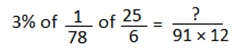a. 7/4
b. 7
c. 3/4
d. 4/7
e. None of the above

a. 7/4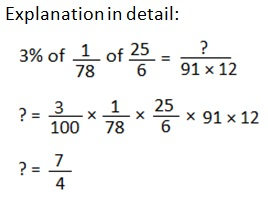Question 4: What value should come in the place of the question mark (?) in the given question?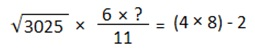a. 1/2
b. 2
c. 1
d. 6
e. None of the above

c. 1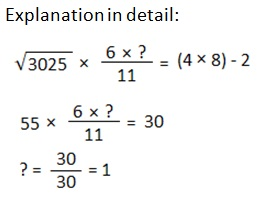Question 5: What value should come in the place of the question mark (?) in the given question?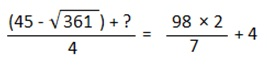a. 124
b. 112
c. 128
d. 102
e. None of the above

d. 102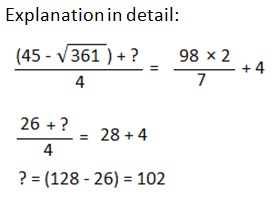Question 6: What value should come in the place of the question mark (?) in the given question?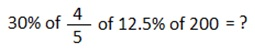a. 5
b. 2
c. 3
d. 6
e. None of the above

d. 6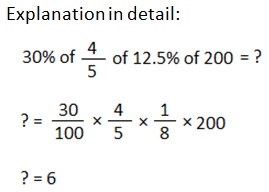Question 7: What value should come in the place of the question mark (?) in the given question?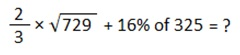a. 44
b. 60
c. 70
d. 54
e. None of the above

c. 70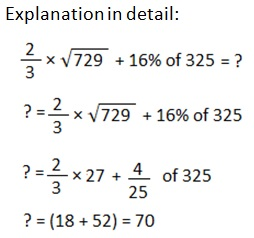Question 8: What value should come in the place of the question mark (?) in the given question?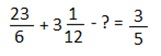a. 379/60
b. 329/40
c. 389/60
d. 479/60
e. None of the above

a. 379/60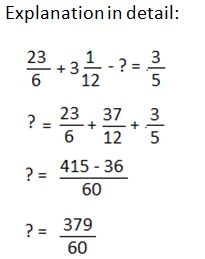Question 9: What value should come in the place of the question mark (?) in the given question?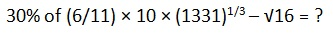a. 18
b. 16
c. 12
d. 14
e. None of the above

d. 14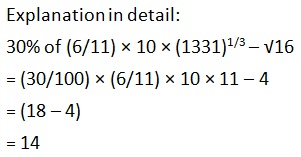Question 10: What value should come in the place of the question mark (?) in the given question?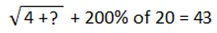a. 3
b. 6
c. 5
d. 4
e. None of the above

c. 5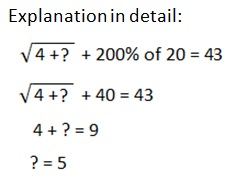IBPS Home

Back to Home